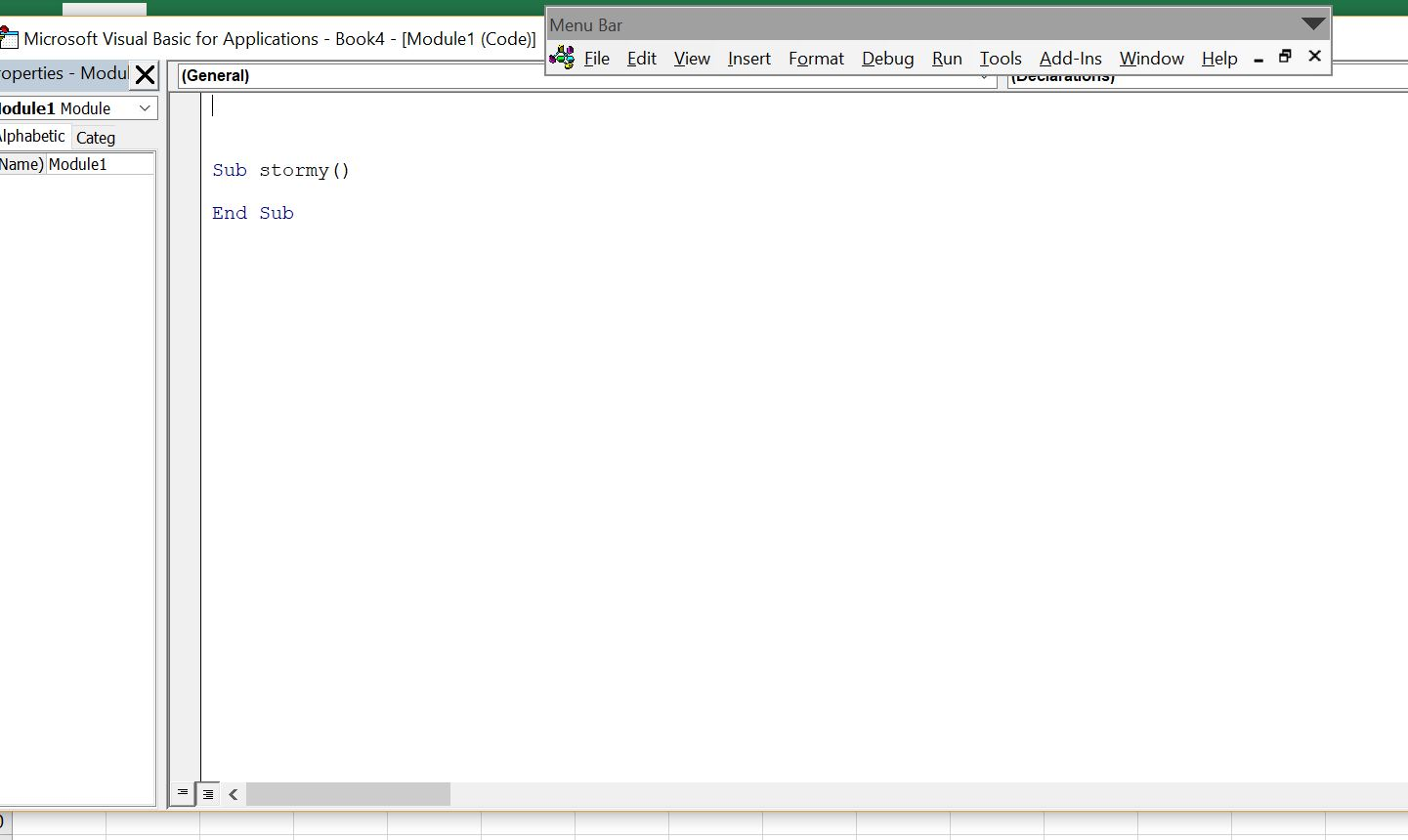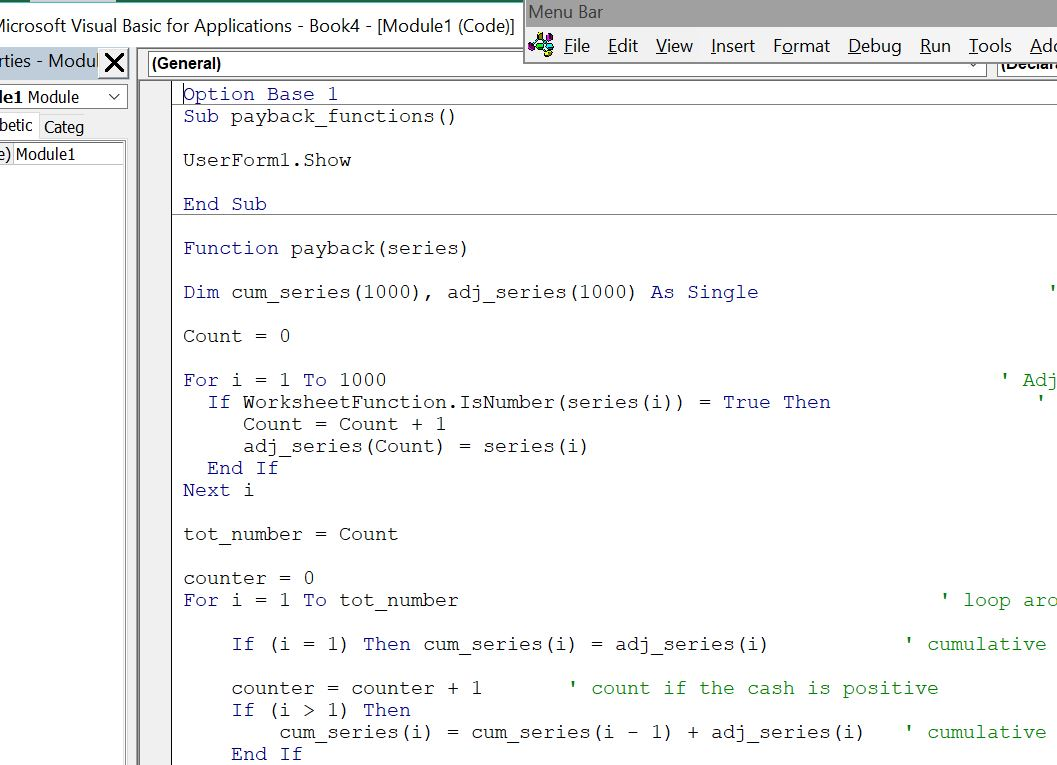# Payback Period

This page shows how you can compute the payback period in excel since there is no payback function.  Of course there are problems with the payback period, but that does not mean it should not even be a function in excel.

This page shows you how to make a payback function in excel with a User Defined Function (UDF).  With the payback function UDF, you can simply enter a function on a series of cash flows and find the payback with percent of month or percent of year with =PAYBACK(cash flows).  Technical details demonstrate how to create the UDF function with VBA code by accumulating cash flow and then computing the percent of the period once the accumulated cash flow changes from negative to positive.

The payback function is heavily critiqued by business schools.  This is snobbish as so many real world decisions are still made by assessing how many years an investment takes to payback.  You can use Match and Index along with adding another line (or column) to find an integer that gives you an approximate payback.  But there is no detailed payback function or discounted payback function in excel, which is amazing.  The file below has a payback function.  You can also just copy the code below into your excel file.  Finally, there is a video that explains how to make a payback function.  The file that contains the payback functions is available for download below.

## Using the Payback UDF

Once you have created the payback UDF function, you can use the PAYBACK function and the DPAYBACK function as shown in the screenshot below. In the example, I have made a simple cash flow and you can demonstrate that if the cash flow after the outflow is 10, the payback period is 10 years.  The example shows that you can click on the entire line to get the payback period.  The discounted payback period counts how many period it takes to repay the cash flow when a discount rate is included for the cash flow.## Inserting the Payback Function into a Your Workbook

After you open the file that is available for download above you can do a few things to get the function into your sheet.  One is to press the button that will produce a user form.  Then, in the text that comes up, just select the VBA code and copy it to your sheet (as shown in the screenshot below the file).  Alternatively, you can press the ALT and F8 key sequence, edit the VBA code, press CNTL, C and then copy it to your file.Once you have copied the VBA code (either from the userform, from pressing ALT, F8 and copying the entire sheet or from copying the code below), you can copy it into your file.  To do this, you can press ALT, F8 to get the VBA screen.  Then put in any name (e.g. Stormy).  Then go to the top of the file and copy the code (CNTL, V).  Some of this is demonstrated in the couple of  screenshots below.  The first screen shot illustrates how to create a new VBA page after you press ALT, F8; the second shows how you should insert a line to put the code at the top of the page; the third shows the results after you copy.After making the new page with you blank macro named Stormy, go above the new macro and enter a couple of blank lines.  When you have entered the blank lines you will press the CNTL, V and copy the code to the top of the page.After you have copied the code into your sheet, you should see OPTION BASE 1 at the top of the page.  Now you are ready to go and the function should work in your workbook.## Creating the Payback Function and Discounted Payback Function

The user defined functions for creating a payback and the discounted payback functions are presented below.  You can see the adjustment required so that an entire row can be used and you can see how the loop works with counting the number of cash flows.

.

```Function payback(series)

Dim cum_series(1000), adj_series(1000) As Single ' dimesion of cumulative cash

Count = 0

For i = 1 To 1000 ' Adjustment for entire row
If WorksheetFunction.IsNumber(series(i)) = True Then ' Only include numbers in new array
Count = Count + 1
End If
Next i

tot_number = Count

counter = 0
For i = 1 To tot_number ' loop around cash flows

If (i = 1) Then cum_series(i) = adj_series(i) ' cumulative cash flow

counter = counter + 1 ' count if the cash is positive
If (i > 1) Then
cum_series(i) = cum_series(i - 1) + adj_series(i) ' cumulative cash flow
End If

If (cum_series(i) > 0) Then ' test when turns to positive
GoTo finished:
End If
Next i
num = i

finished: ' compute payback
If ((cum_series(i) - cum_series(i - 1) <> 0)) Then
factor = -cum_series(i - 1) / (cum_series(i) - cum_series(i - 1)) ' Compute the factor to add to the payback for pct of yr
Else
factor = 0
End If
If (i < series.Count) Then
payback = factor + counter - 2
Else
payback = num
End If

End Function```

.

The function below is very similar, but it includes discounting the cash flow. This discounting can be adjusted if you do not want the initial outflow discounted (in this case you could raise the discount factor to the count minus 1).

```.

Function dpayback(d_rate, series)

Dim cum_series(1000), adj_series(1000), dfactor(1000) As Single ' dimesion of cumulative cash

Count = 0

For i = 1 To 1000 ' Adjustment for entire row
If WorksheetFunction.IsNumber(series(i)) = True Then ' Only include numbers in new array
Count = Count + 1
End If
Next i

tot_number = Count

counter = 0
For i = 1 To tot_number ' loop around cash flows

dfactor(i) = 1 / ((1 + d_rate) ^ counter)

If (i = 1) Then cum_series(i) = adj_series(i) ' cumulative cash flow

counter = counter + 1 ' count if the cash is positive
If (i > 1) Then
cum_series(i) = cum_series(i - 1) + adj_series(i) ' cumulative cash flow
End If

If (cum_series(i) > 0) Then ' test when turns to positive
GoTo finished:
End If
Next i
i = num

finished: ' compute payback
If ((cum_series(i) - cum_series(i - 1) <> 0)) Then
factor = -cum_series(i - 1) / (cum_series(i) - cum_series(i - 1)) ' Compute the factor to add to the payback for pct of yr
Else
factor = 0
End If
If (i < series.Count) Then
dpayback = factor + counter - 2
Else
dpayback = num
End If

End Function```

.

## Video Describing How to Create Payback Functions

If all of the above explanation does not do enough, then you can watch a video on how to create a payback function.